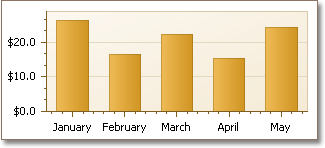# How to: Change the Display Format for Axis Labels (Runtime Sample)

This example demonstrates how you can customize the numerical and date-time options of an axis.

``````using System;
using System.Windows.Forms;
using DevExpress.XtraCharts;
// ...

namespace FormatAxisLabels {
public partial class Form1 : Form {
public Form1() {
InitializeComponent();
}

private void Form1_Load(object sender, EventArgs e) {
// Create an empty chart.
ChartControl chartControl1 = new ChartControl();

// Create a bar series and add points to it.
Series series1 = new Series("Series 1", ViewType.Bar);
series1.Points.Add(new SeriesPoint(new DateTime(2009, 1, 1), new double[] { 26.25 }));
series1.Points.Add(new SeriesPoint(new DateTime(2009, 2, 1), new double[] { 16.52 }));
series1.Points.Add(new SeriesPoint(new DateTime(2009, 3, 1), new double[] { 22.21 }));
series1.Points.Add(new SeriesPoint(new DateTime(2009, 4, 1), new double[] { 15.35 }));
series1.Points.Add(new SeriesPoint(new DateTime(2009, 5, 1), new double[] { 24.15 }));

// Add the series to the chart.

// Hide the legend (if necessary).
chartControl1.Legend.Visibility = DevExpress.Utils.DefaultBoolean.False;

// Set the scale type for the series' arguments and values.
series1.ArgumentScaleType = ScaleType.DateTime;
series1.ValueScaleType = ScaleType.Numerical;

// Cast the chart's diagram to the XYDiagram type, to access its axes.
XYDiagram diagram = chartControl1.Diagram as XYDiagram;

// Define the date-time measurement unit, to which the beginning of
// a diagram's gridlines and labels should be aligned.
diagram.AxisX.DateTimeScaleOptions.GridAlignment = DateTimeGridAlignment.Day;

// Define the detail level for date-time values.
diagram.AxisX.DateTimeScaleOptions.MeasureUnit = DateTimeMeasureUnit.Month;

// Define the custom date-time format (name of a month) for the axis labels.
diagram.AxisX.Label.TextPattern = "{V:MMMM}";

// Since the ValueScaleType of the chart's series is Numerical,
// it is possible to customize the NumericOptions of Y-axis.
diagram.AxisY.Label.TextPattern = "{V:C1}";

// Add a title to the chart (if necessary).
ChartTitle chartTitle1 = new ChartTitle();
chartTitle1.Text = "Axis Scale Types";

// Add the chart to the form.
chartControl1.Dock = DockStyle.Fill;